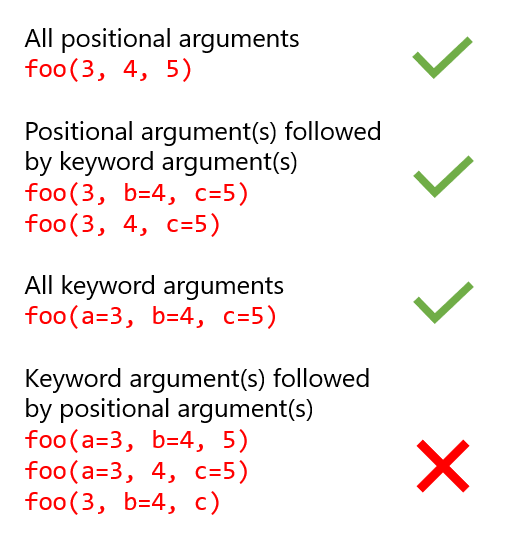# How to Fix: SyntaxError: positional argument follows keyword argument in Python

• Last Updated : 28 Nov, 2021

In this article, we will discuss how to fix the syntax error that is positional argument follows keyword argument in Python

An argument is a value provided to a function when you call that function. For example, look at the below program –

## Python

 `# function``def` `calculate_square(num):``    ``return` `num ``*` `num`` ` ` ` `# call the function``result ``=` `calculate_square(``10``)``print``(result)`

Output

```100
```

The calculate_square() function takes in an argument num which is an integer or decimal input, calculates the square of the number and returns the value.

## Keyword and Positional Arguments in Python

There are two kind of arguments, namely, keyword and positional. As the name suggests, the keyword argument is identified by a function based on some key whereas the positional argument is identified based on its position in the function definition. Let us have a look at this with an example.

## Python

 `# function``def` `foo(a, b, c``=``10``):``    ``print``(``'a ='``, a)``    ``print``(``'b ='``, b)``    ``print``(``'c ='``, c)`` ` ` ` `# call the functions``print``(``"Function Call 1"``)``foo(``2``, ``3``, ``8``)``print``(``"Function Call 2"``)``foo(``2``, ``3``)``print``(``"Function Call 3"``)``foo(a``=``2``, c``=``3``, b``=``10``)`

Output:

```Function Call 1
a = 2
b = 3
c = 8
Function Call 2
a = 2
b = 3
c = 10
Function Call 3
a = 2
b = 10
c = 3```

Explanation:

1. During the first function call, we provided 3 arguments with any keyword. Python interpreted in order of how they have been defined in the function that is considering position of these keywords.
2. In the second function call, we provided 2 arguments, but still the output is shown because of we provided 2 positional argument and the function has a default value for the final argument c. So, it takes the default value into account for the final argument.
3. In the third function call, three keyword arguments are provided. The benefit of providing this keyword argument is that you need not remember the positions but just the keywords that are required for the function call. These keywords can be provided in any order but function will take these as key-value pairs and not in the order which they are being passed.

## SyntaxError: positional argument follows keyword argument

In the above 3 cases, we have seen how python can interpret the argument values that are being passed during a function call. Now, let us consider the below example which leads to a SyntaxError.

## Python

 `# function definition``def` `foo(a, b, c``=``10``):``    ``print``(``'a ='``, a)``    ``print``(``'b ='``, b)``    ``print``(``'c ='``, c)`` ` `    ``# call the function``print``(``"Function Call 4"``)``foo(a``=``2``, c``=``3``, ``9``)`

Output:

```File "<ipython-input-40-982df054f26b>", line 7
foo(a=2, c=3, 9)
^
SyntaxError: positional argument follows keyword argument```

Explanation:

In this example, the error occurred because of the way we have passed the arguments during the function call. The error positional argument follows keyword argument means that the if any keyword argument is used in the function call then it should always be followed by keyword arguments. Positional arguments can be written in the beginning before any keyword argument is passed. Here, a=2 and c=3 are keyword argument. The 3rd argument 9 is a positional argument. This can not be interpreted by the python as to which key holds what value. The way python works in this regards is that, it will first map the positional argument and then any keyword argument if present.

## How to avoid the error – ConclusionMy Personal Notes arrow_drop_up